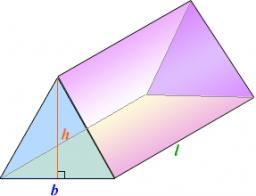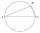# Triangular prism

Plane passing through the edge AB and the center of segmet CC' of regular triangular prism ABCA'B'C', has angle with base 22 degrees, |AB| = 6 cm. Calculate the volume of the prism.

Correct result:

V =  65.45 cm3

#### Solution:

$a = 6 \ cm \ \\ h_1 = \dfrac{ \sqrt3}{2} a = 5.196 \ cm \ \\ \ \\ \tan 22 ^\circ = \dfrac{h/2}{h_1} \ \\ h = 2 h_1 \tan 22 ^\circ = 2 \cdot 5.196 \cdot \tan 22 ^\circ = 4.199 \ cm \ \\ S_1 = \dfrac12 a h_1 = \dfrac12 6 \cdot 5.196 = 15.588 \ cm^2 \ \\ V = S_1 h = 15.588 \cdot 4.199 = 65.45 \ \text{cm}^3 \ \\$We would be very happy if you find an error in the example, spelling mistakes, or inaccuracies, and please send it to us. We thank you!Tips to related online calculators
Pythagorean theorem is the base for the right triangle calculator.
Tip: Our volume units converter will help you with the conversion of volume units.

#### You need to know the following knowledge to solve this word math problem:

We encourage you to watch this tutorial video on this math problem:

## Next similar math problems:

• 3s prismIt is given a regular perpendicular triangular prism with a height 19.0 cm and a base edge length 7.1 cm. Calculate the volume of the prism.
• Triangular prismThe perpendicular triangular prism is a right triangle with a 5 cm leg. The content of the largest wall of the prism is 130 cm2 and the body height is 10 cm. Calculate the body volume.
• ReflectorCircular reflector throws light cone with a vertex angle 49° and is on 33 m height tower. The axis of the light beam has with the axis of the tower angle 30°. What is the maximum length of the illuminated horizontal plane?
• Center traverseIt is true that the middle traverse bisects the triangle?
• MapleMaple peak is visible from a distance 3 m from the trunk from a height of 1.8 m at angle 62°. Determine the height of the maple.
• Right angleIf a, b and c are two sides of a triangle ABC, a right angle in A, find the value on each missing side. If b=10, c=6
• Reference angleFind the reference angle of each angle:The double ladder is 8.5m long. It is built so that its lower ends are 3.5 meters apart. How high does the upper end of the ladder reach?
• Isosceles triangleCalculate the area of an isosceles triangle, the base of which measures 16 cm and the arms 10 cm.
• If theIf the tangent of an angle of a right angled triangle is 0.8. Then its longest side is. .. .
• TreeHow tall is the tree that observed in the visual angle of 52°? If I stand 5 m from the tree and eyes are two meters above the ground.The double ladder shoulders should be 3 meters long. What height will the upper top of the ladder reach if the lower ends are 1.8 meters apart?I have a wall 2m high. I need a 15 degree angle (upward) to second wall 4 meters away. How high must the second wall?Calculate the area of right triangle ΔABC, if one leg is long 14 and its opposite angle is 59°.Find the length of the sides of the triangle KLM if m = 5cm height to m = 4.5 cm and size MKL angle is 70 degrees.Calculate the content of a rectangular trapezoid with a right angle at the point A and if |AC| = 4 cm, |BC| = 3 cm and the diagonal AC is perpendicular to the side BC.Equilateral triangle has a perimeter 36 dm. What is its area?